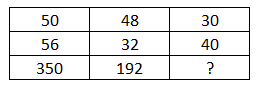Question 23

# Study the given pattern carefully and select the number that can replace the question mark (?) in it.Solution

(50 $$\times 56) \div 8 = 2800/8 = 350$$
(48 $$\times 32) \div 8 = 1536/8 = 192$$
Similarly,
(30 $$\times 40) \div 8 = 1200/8 = 150$$
$$\therefore$$ The correct answer is option A.